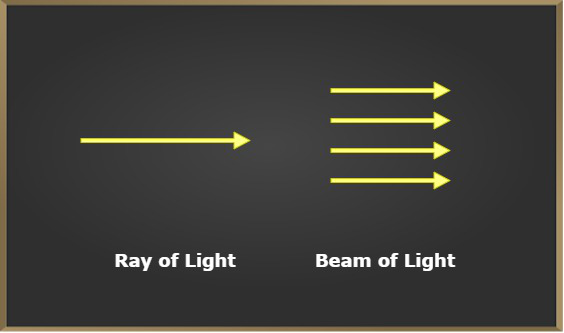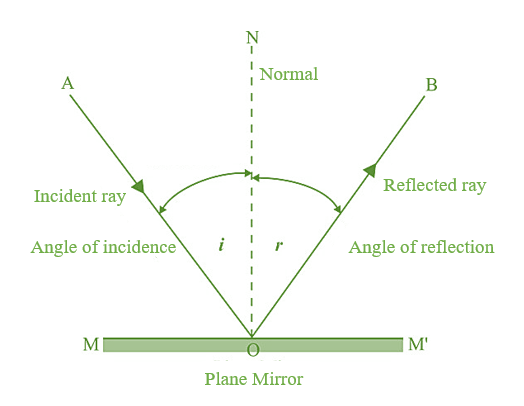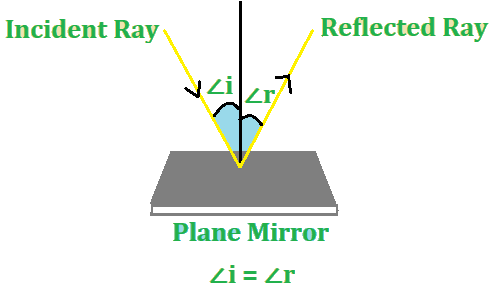Skip to content
Related Articles
Reflection of Light
• Difficulty Level : Expert
• Last Updated : 17 Mar, 2021

Light is something that enables us to see objects around us from the point or source through which it gets reflected or appears to come from. For example, during daytime when sunlight falls on the tree, the tree reflected the light to the observer and so the tree is visible to the observer. However, during the night when there is no light falling on the tree, the tree is not visible properly or in other words, it appears darker. Therefore, it is concluded that sunlight or any other form of light is necessary to view the tree by the observer.The observation of trees in daytime versus in night-time.

### Light

Light is a form of visible energy that causes the sensation of sight in eyes of the observer and makes it to see various objects around it. The light coming out of any object can be its own r may be reflected by other source objects. This way an object can be categorized as two:

1. Luminous objects

The objects which emit their own light or in other words, the objects which are itself the source of the light are called the Luminous objects. The objects like Sun, Halogen bulb, Tubelight, etc emit their own light, therefore, are also called the sources of light or luminous objects.

2. Non- Luminous Objects

Any object which reflects the light falling on it is visible while, if any object neither emits light nor reflects the light falling on it, then the object isn’t visible.  Therefore, the objects which do not emit their own light but reflect the light that falls on them due to other sources of light are called the Non-luminous objects

e.g. Moon doesn’t have its own light but still, it can be seen. This is because the moon reflects the light that fall on it from the Sun, which makes it visible and so the moon is a non-luminous object. Similarly, a book, a table, a tree all are non-luminous objects.

### Some terms related to Light

1. Ray of light: The light traveling in any one direction in a straight line is called a ray of light. A ray of light is a path followed by light energy in a transparent medium. A ray of light is represented by a straight line having the sign of an arrow on it in the direction of propagation of light as shown in the figure.
2. Beam of Light: A group of parallel rays of light emitted by the source of light is called a beam of light.### Nature of Light

The following are the two theories that help to understand the nature of light:

• Wave theory of light: According to wave theory light, light consists of electromagnetic waves and does not require a medium (like solid, liquid or gas) to travel. The wavelength of visible light waves is very small (4 x 10-7 m to 8 x 10-7 m). The speed of light waves is very high (being about 3 x 108 meters per second in vacuum).
• Particle theory of light: According to particle theory of light, light is composed of particles that travel in a straight line at a very high speed. The elementary particle that defines light is called a photon.

### Characteristics of Light

Since light is an important source of energy therefore, there are some characteristics that are needed to be studied, which are:

• Light propagates in a straight line, in a homogeneous transparent medium such a process is called Rectilinear propagation of light.
• It is a form of energy that can be transferred from one medium to another.
• Light is a transverse wave, Light can travel in a vacuum also i.e it does not require any medium to propagate. The speed of light in air is equal to 3 x 108 m/s.
• The speed of light can change when it travels from one medium to another.
• The wavelength (λ) of light changes when it travels from one medium to another medium.
• The frequency (f) of the light wave never changes, it remains the same in all mediums.
• Light shows dual nature i.e it behaves like a particle as well as a wave also.
• Three phenomenons can be possible when light interacts with an object in a medium: Reflection, refraction; absorption.
• According to current scientific theories, no material particle can travel at a speed greater than that of light in vacuum.

### Reflection of Light

The process of returning or bouncing back of the light rays to the same medium after striking a surface is called reflection of light. The surface which reflects the light is known as a reflector. The polished metal surfaces are good reflectors. However, silver metal is the best reflector. Even water surfaces, waxed surfaces, and glazed paper act as reflectors. The most commonly used reflector is a looking glass or a plane mirror.

Consider a ray of light AO incident on the plane mirror MM’ at O, which after reflection from the mirror bounce back in the same medium followed the path OB. The ray OA and OB are called the incident ray and the reflected ray. Suppose ON is the normal of the plane mirror, then the angle between AO i.e incident ray, and the normal is called the angle of incidence while the angle between OB i. reflected ray and the normal is called the angle of reflection. Each of the terms related to the reflection located on the figure shown below:Normal: A perpendicular drawn to the reflecting surface at point O (known as the point of incidence) is called the normal to the reflecting surface.

Incident Ray: A ray of light that falls on the reflecting surface from a light source or an object is called the incident ray.

Reflected Ray: A ray of light that arises from the reflecting surface after reflection from it is called a reflected ray

Angle of incidence: The angle between the incident ray and normal to the point of incidence on the reflecting surface is known as incident angle or Bugle of incidence. It is denoted byAngle of reflection: The angle between the reflected ray and the normal to the point of incidence on the reflecting surface is known as the angle of reflection. It is denoted by### Laws of Reflection

The reflection of light from a surface obeys certain laws called Laws of reflection, stated as:

First Law of reflection: According to this law: The incident ray, the reflected ray and the normal to the reflecting surface at the point of the incident, all lie on the same plane.

Second Law of reflection: According to this law: The angle of reflection is always equal to the angle of incident.

Let angle of incident is equal toand angle of reflection isthen according to the law of reflection of light,### Sample Problems

Example 1: If angle of incidence of a ray of light is 35°, calculate angle of reflection.

Solution:

Given, angle of incidence=35°

According  to law of reflection,

∠i=∠r

∠r=35°

Hence angle of reflection is 35°.

Example 2: An incident ray makes an angle of 45° with the surface of a plane mirror, calculate angle of reflection.

Solution:

We will first calculate the angle of incidence. In this case, the incident ray makes an angle of 45° with the surface of the mirror,

The angle of incidence is the angle between incident ray and normal.

So, in this case, the angle of incidence will be 90° – 45°= 45°.

Since the angle of incidence is 45°.

According  to law of reflection,

∠i=∠r

Hence angle of reflection is 45°.

My Personal Notes arrow_drop_up